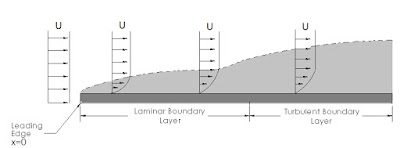### Boundary Layer Part TwoTurbulent Taylor-Couette flows with endcap rings

This is the second part of boundary layer miniseries.

The Reynolds number can be used to predict a flow pattern. The onset of very fascinating flow patterns, for example vortex streets (Tutorial here) can be detected with the use of Reynolds numbers.

The Reynolds number is the ratio between inertial and viscous forces. A low Reynolds number indicates that inertial forces are overwhelmed by viscous forces. Conversely a high Reynolds number indicates that inertial forces are driving the flow. We can distinguish three principal flow patterns: laminar, transitional and turbulent.

We have a laminar flow when the fluid flows in parallel layers, each layer having little interaction with the adjacent layer. In a laminar flow it is possible to distinguish parallel flowing streamlines. An extreme example of laminar flow is shown on the following video.

Video 1 - Laminar flow twisting and untwisting

This flow has a very low Reynolds number, well below unity. As the flow is strongly laminar the operator can rewind the system to a state visually identical to the original state

Turbulent flow is characterized by an apparent randomness, circulation, eddies and high Reynolds number.

A transitional flow is somewhat in between the two previous cases: some parts of the fluid can have a laminar behavior and some others a turbulent-like behavior. Determining the Reynolds number for each pattern in the transtional flow is not trivial, but fortunately for many notable cases there is an approximate math model. In the next video a complete transition between laminar and turbulent flow into a pipe is shown.

Video 2 – Transition between patterns

In the relevant literature, one of the most well-studied boundary layer patterns is the one formed by a flow over a flat plate. Under some assumptions, the boundary layer expression can be analytically found, for example there is the Blasius solution. Have a look at Figure 1. One important thing to observe is that the boundary layer is developing along the plate $$x$$ coordinate, on the horizontal direction of the image. The Reynolds number expression is $$Re=\rho Ux/\mu$$ and $$x=0$$ corresponds to the leading edge of the plate or the beginning of the boundary layer itself. Initially, the flow is laminar and as $$x$$ increases the Reynolds number increases as well. At some point, the boundary layer transforms into a transitional pattern and finally ends with a turbulent configuration. If one is able to increase $$x$$ at will, the flow will become turbulent sooner or later. Depending on many factors, like fluid type and plate roughness the transition to turbulent flow will happen at a different Reynolds number. A plausible interval of values is from 1e5 to 3e6.Figure 1 – Boundary layer over a flat plate

The larger the x-coordinate of the section we examine is, the wider the corresponding boundary layer will be. Given a section at a coordinate $$x$$, we observe that the thickness of the corresponding boundary layer decreases as the free stream speed increases. We can observe the relationship between freestream speed and boundary layer thickness on the following video.

Video 3 - Boundary layer experiment water tube

In the video, the transition to turbulent pattern is triggered by an aerodynamic obstacle. Pressure gradients can cause transitions of boundary layers. Pressure gradients are likely to occur on external air temperature probes inside the diffuser section. You can see the geometry of a Kiel probe here

In the general case, boundary layer shape is time and position dependent and thus no overall closed form solution is available.

In the next article we will investigate in more detail the simplest ways that pressure gradients affect boundary layers and we will examine a numerical example.